HOME COURSES PREVIEW REVIEW ABOUT CONTACT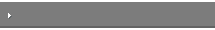Toll-Free Info & Ordering M-F: 9am-5pm (PST): (877) RAPID-1024/7 Technical SupportQUICK TOURMember Login: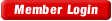Rapid Courses Catalog Mathematics in 24 Hours Chemistry in 24 Hours Biology in 24 Hours Physics in 24 HoursMath Survival Weekly
Get the insider's tips and tricks in how to survive your math course and ace the next test. Subscribe the Web's only math weekly newsletter for students and learn:
- How to Study Math Effectively
- How to Take Math Courses Strategically
- How to Solve Math Problems Systematically
- How to Score High in Math Exams
- How to Master Math Rapidly

Enter your name and email below and get started today!Math Study Lounge These study sheets are for quick review on the subjects. Refer to our rapid courses for comprehensive review.     - Getting Started with Algebra     - Geometry Basics     - How to Solve Math Problems     - Trigonometry Quick Review     - Statistics At-A-Glance     - Calculus PreviewHome » Geometry Basics

Key Geometry Terms

• Angle - either the figure formed by two rays starting from a common point or the measure of such a figure.
• Complimentary Angles - a pair of angles whose sum is 90˚.
• Supplementary Angles - a pair of angles whose sum is 180˚.
• Congruent Angles - angles with the same angle measurement.
• Vertical Angles - angles at an intersection of two lines that are not adjacent to each other.
• Interior Angles - pair of angles that are in the interior of a polygon, OR angles inside or between two parallel lines.
• Exterior Angles - pair of angles formed by the side of a polygon and an adjacent, extended side OR angles on the outside of two parallel lines.
• Circle - a figure in which all points are the same distance from the center, that distance being known as the radius.
• Pi - ratio of the circumference to the diameter of a circle.  Pi is denoted by the Greek symbol “π” and is approximately 3.14159, though it is widely known to continue to an infinite number of decimals and thus, is irrational.
• Pythagorean Theorem - the sum of the squares for the legs of a right triangle equal the square of the hypotenuse
• Special Right Triangles - right triangles where certain relationships always hold true.  These relationships, when recognized, are useful since they reduce sometimes complex work to solving a proportion or even mental math.
• Regular polygon - a geometric figure with three or more sides in which all interior angles are congruent.
• Area - size of a planar region or surface of a solid.  Areas are measured in “square” units.
• Volume - amount of space taken up by or enclosed by an object.  Volumes are measured in “cubic” units.
• Degree - unit of angle measurement equal to 1/360 of a circle’s circumference.
• Radian - unit of angle measurement that is the ratio ofthe circle arc cut off by an angle to the central angle.
• Sine - on a unit circle, the “y” coordinate for any intersection between a standard angle and the circle.  In a right triangle, the ratio of the length of the opposite side to the length of the hypotenuse.
• Cosine - on a unit circle, the “x” coordinate for any intersection between a standard angle and the circle.  In a right triangle, the ratio of the length of the adjacent side to the length of the hypotenuse.
• Tangent - the ratio of sine to cosine or, in a right triangle, the ratio of the length of the opposite side to the length of the adjacent side.
• Secant - reciprocal of cosine (sec θ = 1 / cos θ).
• Cosecant - reciprocal of sine (csc θ = 1 / sin θ).
• Cotangent - reciprocal of tangent (cot θ = 1 / tan θ).
• Vector - a directed line segment.  The length of the segment indicates magnitude or amount in a measurement and the direction of the segment, indicated with an arrow, indicates the direction of the measurement
Basic Rules of Angles
• Vertical angles are always congruent!
• The alternate interior angles of parallel lines cut by a transversal are congruent.
• The alternate exterior angles of parallel lines cut by a transversal are congruent

Shapes and Figures

• Pythagorean Theorem: a2 + b2 = c2.
• 3 : 4 : 5 right triangle: legs that are 3 and 4 units long resulting in a hypotenuse that is 5 units long.
• 5:12:13 right triangle: legs that are 5 and 12 units long resulting in a hypotenuse that is 13 units long.
• 30-60-90: when the angles are 30, 60 and 90 degrees, the sides are in ratios of 1 : √3 : 2 .
• 45-45-90: when the angles are 45, 45 and 90 degrees, the sides are in ratios of
√2 : √2 : 2 .
• All regular polygons: if “n” is the number of sides, then the sum of the interior angles may be found by using: ( n – 2 ) ( 180˚ )

Area and Volume Formulas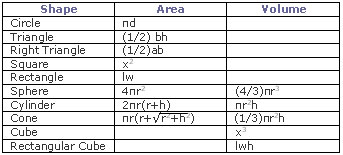• It is possible to approximate the area of a figure by using smaller figures, such as triangles and rectangles, to approximate the figure.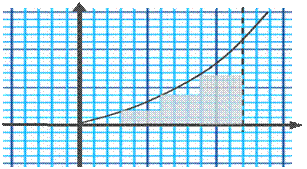• We can break the figure into a number of rectangles and find the area of each:
3x2 + 3x4 + 3x6.5 + 3x11 = 70.5 square units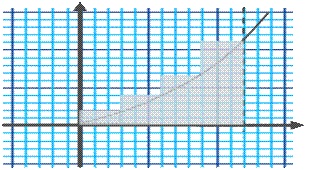• This may be done alternatively:
3x2 + 3x4 + 3x6.5 = 37.5 square units
• We can average this and the previous result to get a better approximation than either alone: 59 square unit
Would you like to study the entire math course in 24 hours? Master all important concepts and learn how to solve problems. Super review your upcoming exams with our cheat sheets. Get started with out rapid courses now!
Calculus
Introductory Statistics

To quickly learn about our math rapid learning system, view the movie:
 ©2014 Rapid Learning Center. Privacy Policy | Disclaimer Chemistry Survival Publishing, Biology Survival Publishing and Physics Survival Publishing are the divisions of Rapid Learning Inc.